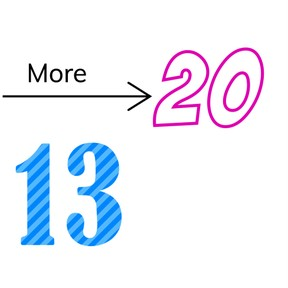Comparing numbers to 20

# Comparing numbers to 20

Comparing numbers to 208,000 schools use Gynzy92,000 teachers use Gynzy1,600,000 students use Gynzy

## General

Students learn to compare numbers from 1 to 20 and know when a number is more or less.

1.NBT.B.3

## Introduction

The interactive whiteboard shows an image of twelve colored pencils and eleven students. Ask the class if there are enough colored pencils for the students. Ask students to explain how they got to their answer. Next show images of groups of balls from 1 to 10. Ask which groups are less than four. Ask students to explain how they got their answer.

## Instruction

Show the number line on the board and show students how one number is more than another by indicating how place on the number line shows when a number is more or less. To explain set two groups of blocks on the table at the front. Count the number of blocks in each group and write the amount of the group down. Ask the class which pile has more blocks. Give a few more examples of more and less using the number line. As a class, compare a few numbers. Tell students that they can use the number line as support.

Check that students can compare numbers to 20 by asking the following questions:
- When is one number more than another number?
- How do you know if a number is more or less?
- Which number(s) are more than 14? How do you know?
- Write down a number that is less than 16.

## Quiz

Students practice with the terms of more and less in combination with numbers. Next, they also practice with the terms most and least.

## Closing

Discuss with students that it is important to be able to compare numbers, to help you learn for example what is more or less expensive. Check that students know that they can use the number line to determine their answers. Check that they know how to compare numbers by having students compare a number. Next, they must say a number that is more or less than the number shown on the ball on the interactive whiteboard.

## Teaching tips

Students who have difficulty comparing numbers can practice with the number line. By placing the numbers on the number line, they can see which numbers come first and which come later, showing which are more or less.

## Instruction materials

Blocks

### The online teaching platform for interactive whiteboards and displays in schools

• Save time building lessons

• Manage the classroom more efficiently

• Increase student engagement